Anúncio

# Family fun night.pptx

21 de Mar de 2023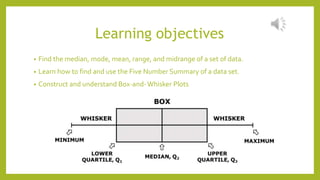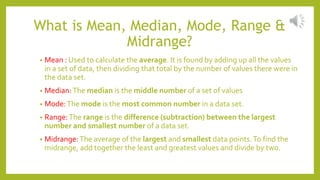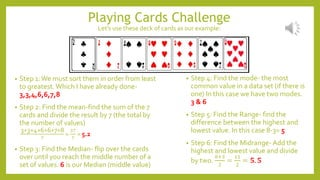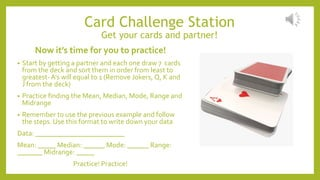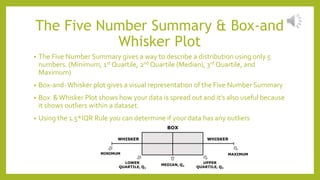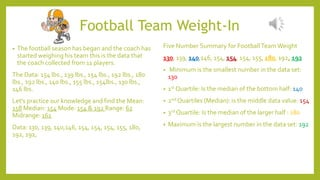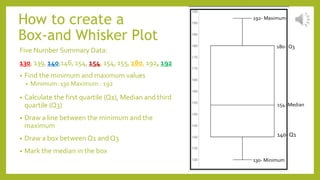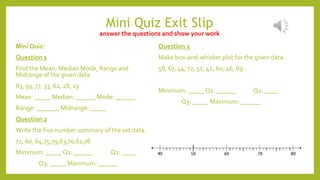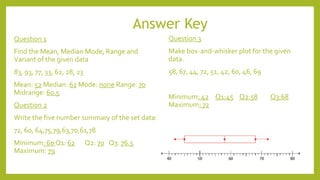1 de 11
Anúncio

### Family fun night.pptx

1. FAMILY FUN NIGHT Anilu Mendiola | Math 115 | Final Project
2. Learning objectives • Find the median, mode, mean, range, and midrange of a set of data. • Learn how to find and use the Five Number Summary of a data set. • Construct and understand Box-and-Whisker Plots
3. What is Mean, Median, Mode, Range & Midrange? • Mean : Used to calculate the average. It is found by adding up all the values in a set of data, then dividing that total by the number of values there were in the data set. • Median:The median is the middle number of a set of values • Mode:The mode is the most common number in a data set. • Range:The range is the difference (subtraction) between the largest number and smallest number of a data set. • Midrange:The average of the largest and smallest data points.To find the midrange, add together the least and greatest values and divide by two.
4. Playing Cards Challenge Let’s use these deck of cards as our example: • Step 1:We must sort them in order from least to greatest.Which I have already done- 3,3,4,6,6,7,8 • Step 2: Find the mean-find the sum of the 7 cards and divide the result by 7 (the total by the number of values) 3+3+4+6+6+7+8 7 = 37 7 = 5.2 • Step 3: Find the Median- flip over the cards over until you reach the middle number of a set of values. 6 is our Median (middle value) • Step 4: Find the mode- the most common value in a data set (if there is one) In this case we have two modes. 3 & 6 • Step 5: Find the Range- find the difference between the highest and lowest value. In this case 8-3= 5 • Step 6: Find the Midrange- Add the highest and lowest value and divide by two. 8+3 2 = 11 2 = 𝟓. 𝟓
5. Card Challenge Station Get your cards and partner! Now it’s time for you to practice! • Start by getting a partner and each one draw 7 cards from the deck and sort them in order from least to greatest- A’s will equal to 1 (Remove Jokers, Q, K and J from the deck) • Practice finding the Mean, Median, Mode, Range and Midrange • Remember to use the previous example and follow the steps. Use this format to write down your data Data: _________________________ Mean: _____ Median: ______ Mode: ______ Range: _______ Midrange: _____ Practice! Practice!
6. The Five Number Summary & Box-and Whisker Plot • The Five Number Summary gives a way to describe a distribution using only 5 numbers. (Minimum, 1st Quartile, 2nd Quartile (Median), 3rd Quartile, and Maximum) • Box-and-Whisker plot gives a visual representation of the Five Number Summary • Box &Whisker Plot shows how your data is spread out and it’s also useful because it shows outliers within a dataset. • Using the 1.5*IQR Rule you can determine if your data has any outliers
7. Football Team Weight-In Five Number Summary for FootballTeamWeight 130, 139, 140,146, 154, 154, 154, 155, 180, 192, 192 • Minimum is the smallest number in the data set: 130 • 1st Quartile: Is the median of the bottom half: 140 • 2nd Quartiles (Median): is the middle data value: 154 • 3rd Quartile: Is the median of the larger half : 180 • Maximum is the largest number in the data set: 192 • The football season has began and the coach has started weighing his team this is the data that the coach collected from 11 players. The Data: 154 lbs., 139 lbs., 154 lbs., 192 lbs., 180 lbs., 192 lbs., 140 lbs., 155 lbs., 154lbs., 130 lbs., 146 lbs. Let’s practice our knowledge and find the Mean: 158 Median: 154 Mode: 154 & 192 Range: 62 Midrange: 161 Data: 130, 139, 140,146, 154, 154, 154, 155, 180, 192, 192,
8. Five Number Summary Data: 130, 139, 140,146, 154, 154, 154, 155, 180, 192, 192 • Find the minimum and maximum values • Minimum: 130 Maximum : 192 • Calculate the first quartile (Q1), Median and third quartile (Q3) • Draw a line between the minimum and the maximum • Draw a box between Q1 and Q3 • Mark the median in the box 154- Median 130- Minimum 192- Maximum 140- Q1 180- Q3 How to create a Box-and Whisker Plot
9. Team Group Station Now it’s time for you to practice! • Start by getting a scale and your group of 4-5 . Each one will weight themselves and gather the data.Then you will each be responsible of creating your groups Box-and-Whiskers plot and finding the following data: • Student 1: • Student 2: • Student 3: • Student 4: • Student 5: Data: _________________________ Mean: _____ Median: ______ Mode: ______ Range: _______ Midrange: _____ Write the five number summary of your group data: Minimum: _____Q1: ______ Q2: ____ Q3: _____ Maximum: ______ Make box-and-whisker plot for the given data.
10. Mini Quiz Exit Slip answer the questions and show your work Mini Quiz: Question 1 Find the Mean, Median Mode, Range and Midrange of the given data 83, 93, 77, 33, 62, 28, 23 Mean: _____ Median: ______ Mode: ______ Range: _______ Midrange: _____ Question 2 Write the five number summary of the set data: 72, 60, 64,75,79,63,70,61,78 Minimum: _____Q1: ______ Q2: ____ Q3: _____ Maximum: ______ Question 3 Make box-and-whisker plot for the given data. 58, 67, 44, 72, 51, 42, 60, 46, 69 Minimum: _____Q1: ______ Q2: ____ Q3: _____ Maximum: ______
11. Answer Key Question 1 Find the Mean, Median Mode, Range and Variant of the given data 83, 93, 77, 33, 62, 28, 23 Mean: 52 Median: 62 Mode: none Range: 70 Midrange: 60.5 Question 2 Write the five number summary of the set data: 72, 60, 64,75,79,63,70,61,78 Minimum: 60 Q1: 62 Q2: 70 Q3: 76.5 Maximum: 79 Question 3 Make box-and-whisker plot for the given data. 58, 67, 44, 72, 51, 42, 60, 46, 69 Minimum: 42 Q1:45 Q2:58 Q3:68 Maximum: 72
Anúncio